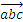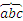# Math

In this subclause, every mathematical expression is called an equation, even if the expression is merely a string of variables or a single object such as a fraction. In XML, an equation is called OMath. A Math Paragraph is a group of one or more equations separated by soft carriage returns; that is, they are separate equations that comprise a single paragraph. A Math Paragraph carries its own justification that can be separate from the justification of the paragraph that contains it. Different equations within a Math Paragraph cannot have different types of justification.

Equations can be Display (the only text on the line) or Inline (on a line with text outside of the equation). The Display vs. Inline state is not specified by this standard; instead, a text processor determines how to format Display and Inline equations. Display and Inline equations innately carry different formatting characteristics; Inline equations consume less vertical space so as not to disrupt line spacing with adjacent lines. This means, for example, reducing the size of fractions and n-ary objects that grow.

The following subclauses introduce each of the objects (also called functions) that comprise the majority of the Equations schema. As a text processor and not a calculation engine, when converting equations into XML representation, more attention is given to the layout and appearance than to the mathematical meaning of the expressions. That is,andare represented with the same object, although they carry different mathematical meanings, because both consist of text paired with a stretching character. Similarly,andare represented as the same object. Though mathematically they have different meaning, their layout is similar.

Although the functionality described in this clause is purely about the appearance of equations, other markup defined in this Office Open XML Standard provides independent functionality enabling calculation of mathematical expressions. Formulas in SpreadsheetML (§3.15.1) and Fields in WordprocessingML (§2.17.1) are two examples.